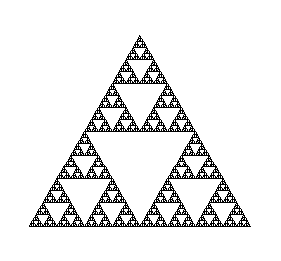First, we have a procedure that takes one triangle as input, and returns three triangles with a side length of half the original. This is the basic generation step for the gasket.
Note that it returns 3 triangles, NOT a list of 3 triangles.

```OneToThree:=proc(tri)
local mid1, mid2, mid3;

mid1:=[(tri+tri)/2,(tri+tri)/2];
mid2:=[(tri+tri)/2,(tri+tri)/2];
mid3:=[(tri+tri)/2,(tri+tri)/2];

RETURN( [tri, mid1,   mid3,  tri  ],
[mid1,   tri, mid2,  mid1   ],
[mid2,   tri, mid3,  mid2   ]);
end:
```

Now we write gasket, which takes a list of triangles as input, and applies OneToThree to each triangle. It then repeats the process on THAT list, numsteps times.

```gasket:=proc(start,numsteps)
local triangles, i, k;

triangles:=start;
for i from 1 to numsteps do
triangles:=[seq(OneToThree(triangles[k]), k=1..nops(triangles))];
od;

RETURN(triangles);
end:

```

Finally, we have a handy utility routine that allows us to plot several disjoint curves (our triangles) on the same plot, with no axes.

```PlotCurves:= proc(curvelist)
local i;
plots[display](
PLOT( seq(CURVES(curvelist[i]),i=1..nops(curvelist))),
axes=none,scaling=constrained);
end:
```

FirstTri is an equlateral triangle of side length 2, which we use for our "level 0" gasket.

```FirstTri := evalf([ [0,0], [1,sqrt(3)], [2,0], [0,0] ]):
PlotCurves([FirstTri]);
```We can now refine this a few times to get a level 3 gasket:

```l3:= gasket([FirstTri], 3):
PlotCurves(l3);
```If we want, we can use the previous figure (l3) as input, and refine it further. Thus, the following should give us a level 6 gasket:

```l6:= gasket(l3 , 3):
PlotCurves(l6);
```Had we been so inclined, we could have written the gasket procedure recursively instead of iteratively. Here is one way to do that:

```cheese:=proc(trilist,n)
local i;

if (n<=0) then
RETURN(op(trilist));
else
RETURN( seq(cheese([OneToThree(trilist[i])], n-1),
i=1..nops(trilist)));
fi;

end:
```

This works by using OneToThree to triple each triangle in the list, as gasket does. It then calls itself on the resulting list of 3 triangles, asking for one level less of decoration. When the number of levels to go gets to zero, the input is returned (stripped of one level of nesting).

As a consequence, notice that the output is a bunch of triangles, NOT a list of triangles as in gasket. This means that we have to wrap the result in brackets before we can feed it into PlotCurves.

The procedure cheese is not a better way than gasket to make the Sierpinski gasket; they are just two different ways of doing the same thing.
Here is the result.

```PlotCurves([cheese([FirstTri],6)]);
```